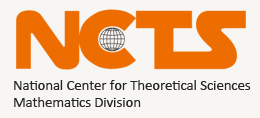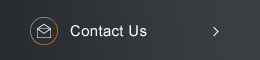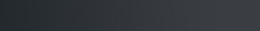Sponsored by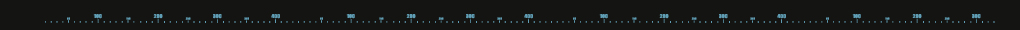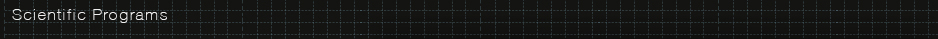Differential Equations and Stochastic Analysis

### Program Committee:

Jung-Chao Ban (NCCU), Chi-Hin Chan (NCTU), Long-Chi Chern (NCCU), Chun-Hsiung Hsia (NTU, committee chair),
Chun-Yen Shen (NTU), Kun-Chien Wu (NCKU).

Description of program:

The study of differential equations is one of the most traditional fields of mathematics in Taiwan. The NCTS Topical Program Differential Equations and Stochastic Analysis also aims to incorporate interdisciplinary research as one of its priorities, and its four main directions are: partial differential equations, dynamical systems, stochastic analysis and harmonic analysis. Partial differential equations are one of the most widely adopted mathematical tools in modern sciences. Members of this NCTS Topical Program group are interested in both the developments of the mathematical theory and scientific applications. This includes, for example, synchronization problem, kinetic theory, elliptic partial differential equations, fluid dynamics, inverse problem and reaction-diffusion equations. Interests of the research groups in dynamical systems include bifurcation theory and chaotic systems, ergodic theory, fractal geometry, arithmetic dynamical systems, and complex dynamical systems. The research interests of Harmonic analysis group include two weights problems for singular integrals, additive combinatorics and their connections to geometric measure theory. Probability theory also plays an important role in many other areas of mathematics, such as partial differential equations, analysis, and combinatorics. It provides the theoretical basis for statistics. In stochastic analysis at the NCTS, focus is placed on statistical mechanics, mathematical biology, finance, theoretical computer science, branching processes and SPDE.

Affiliated members:

Differential equations:

Chi-Hin Chan (NCTU), Chiun-Chuan Chen (NTU), I-Kun Chen (NTU), Zhi-You Chen (NCUE),
Ching-Hsiao Cheng (NCU), Jann-Long Chern (NTNU), Jong-Shenq Guo (TKU), Chun-Hsiung Hsia (NTU),
Hsin-Yuan Huang (NCTU), Hung-Wen Kuo (NCKU), Dong-Ho Tsai (NTHU), Jenn-Nan Wang (NTU),
Kun-Chien Wu (NCKU)

Dynamical systems:

Jung-Chao Ban (NCCU), Chih-Hung Chang (NUK), Kuo-Chang Chen (NTHU), Cheng-Hsiung Hsu (NCU)

Probability:

Guan-Yu Chen (NCTU), Long-Chi Chen (NCCU), Hao-Wei Huang (NSYSU), Chii-Ruey Hwang (AS),
Gi-Ren Liu (NCKU), Yuan-Chung Sheu (NCTU)

Analysis:

Chin-Cheng Lin (NCU), Chun-Yen Shen (NTU), Daniel Spector (OIST)Events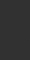2021/04/21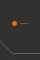Global Dynamics on One-dimensional Excitable Media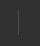Abstract: The FitzHugh–Nagumo system has been studied extensively for several decades. ...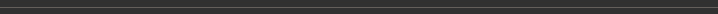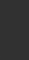2021/04/21The Property of Collision Operator (VIII)Abstract: In this series of talks, we shall follow Chapter 3 of the book of Robert Glassey:Th...2021/04/20Numerical Propagation Speed of Evolution Solution in Lotka-Volterra ModelAbstract: We study Cauchy problem to scaled Lotka-Volterra model with 2 species :   ...VisitorsLien-Yung KaoGeorge Washington Universitylkao[AT]gwu.eduMarch 18 - May 31, 2021Yin-Ting LiaoBrown Universityyin-ting_liao[AT]brown.eduJanuary 1 - June 30, 2021Russell LodgeIndiana State Universityressell.lodge[AT]indstate.eduMay 26 - August 3, 2021(C) 2021 National Center for Theoretical Sciences